Resolution, Prolog

c) 2000, Lee Spector

lspector@hampshire.edu

Resolution in the Propositional Calculus

A proof method that can be extended for the predicate calculus

A single rule of inference -- the resolution principle: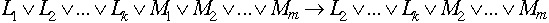where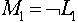.

Example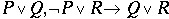Proofs using the resolution principle in the propositional calculus

Assert all premises and the negation of the desired conclusion. Then try to derive the empty clause (which represents a contradiction: it is a disjunction with no disjuncts, so it cannot be true).

Example

Premises: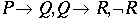Clause form: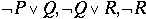Desired conclusion: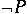Proof

label       clause           where from

C1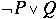Premise

C2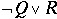Premise

C3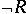Premise

C4       P       Negation of conclusion

C5       Q       Resolve C1 with C4

C6       R       Resolve C2 with C5

C7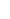*       Resolve C3 with C6

Resolution in the predicate calculus requires three enhancements:

1) A more elaborate procedure for converting statements into clause form.

2) A more elaborate procedure for detecting of "complementary pairs" (unification)

3) Unification variable bindings must be substituted in resolvents.

To convert a list of statements into clause form:

1. Eliminate implications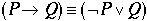2. Move negations down to the atomic formulas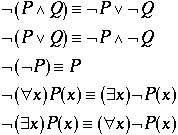3. Purge existential quantifiers using Skolem functions taking one argument for each universally quantified variable in the scope (use a Skolem constant if there are none).

Example: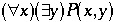can be replaced with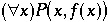4. Rename variables if necessary (each quantified variable should have a different name).

5. Move the universal quantifiers to the left.

6. Move the disjunctions down to the literals.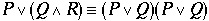7. Eliminate the conjunctions (list each conjunct as a separate formula, using whatever quantifiers correspond to the variables in the formula).

8. Rename variables again, if necessary.

9. Purge the universal quantifiers.

Unification

Unification is a pattern match between two or more expressions, all of which may contain variables.

Often there are many variable substitutions that will unify a set of expressions. In general we want the most general unifier (MGU).

A variable may be replaced by:

* a constant

* a variable

* a function expression as long as it doesn't contain the variable.

Example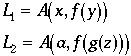The MGU for {L1, L2} is {(a,x), (g(z),y)}.

Another, less general unifier is {(a,x), (g(b),y), (b,z)}

Tanimoto defines a 2-term unification function. Here is the result of his TEST function:

Result of UNIFY on (P X (F A)) and (P B Y) is (((F A) Y) (B X)).

Result of UNIFY on (P (F X) (G A Y)) and (P (F (H B)) (G X Y)) is

NOT-UNIFIABLE.

Result of UNIFY on (P X) and (P (F X)) is NOT-UNIFIABLE.

Result of UNIFY on (P X (F Y) X) and (P Z (F Z) A) is

((A Z) (A Y) (A X)).

Result of applying U to (P X (F Y) X) is (P A (F A) A).

Result of applying U to (P Z (F Z) A) is (P A (F A) A).

An example of resolution in the predicate calculus (Tanimoto)

Premises: Any prime number other than 2 is odd. The square of an odd number is odd. The number 7 is a prime. The number 7 is different from 2.

Prove: The square of 7 is odd.

Predicates:

P(x): x is prime

O(x): x is odd

E(x,y): x = y

s(x) = x2

Premises in predicate calculus: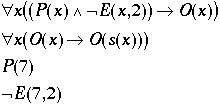Negation of the goal: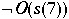Proof

label       clause                         how derived

C1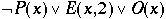First premise in clause form

C2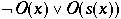Second premise in clause form

C3       P(7)             Third premise in clause form

C4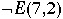Fourth premise in clause form

C5Negated conclusion

C6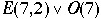C1, C3 (x=7)

C7       O(7)             C6, C4

C8       O(s(7))             C7, C2 (x=7)

C9       *                   C8, C5

This proof was guided by human intuition. In general there will be very large numbers of possible resolutions at every step, and care must be taken to ensure that progress is made toward the derivation of a null clause.

Strategies

Set of Support: give priority to resolvents derived from the clauses that express the negation of the goal.

Linear Format: insist that each step build on the results of the last.

Unit preference: prefer to resolve with unit (1-literal) clauses.

Horn Clauses

At most one of the literals in each clause is unnegated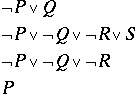These can be rewritten: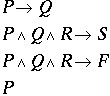They can also be written in "goal-oriented" format: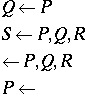Prolog

Prolog is a programming language based on logic.

A Prolog program consists of Horn clauses.

Programming in PROLOG is quite different from programming in LISP

(or in C, or Pascal, etc.).

3 critical ideas behind Prolog:

1) a uniform knowledge base

2) unification of logic variables

3) automatic backtracking

In most programming languages we program by writing procedures. Procedures tell the computer "what to do" when they are called. Such languages are called procedural languages.

In Lisp we write functions. Functions can be thought of as procedures. If you think of the function telling the computer "what to return" rather than "what to do", then you are thinking of Lisp as a functional language rather than as a procedural language.

In Prolog, we program by stating facts and rules. Facts and rules don't tell the computer what to do or what to return -- they merely state truths. We can also ask Prolog to try to prove statements based on what it knows. We don't tell Prolog how to prove it -- Prolog takes care of that by itself. When viewed in this light, Prolog is considered a declarative language. Full implementations of Prolog also include procedural elements, but the flavor of Prolog is decidedly declarative.

Tanimoto provides a "mock Prolog" written in Lisp.

Examples

```;;; Here we declare certain symbols to be variables.
;;; Others are assumed to be functions or constants.
(defvar *known-variables*
'(u v w x y z wine entree lst lst2 r) )

;;; database of clauses for example 1:
(setf database1 '(
((grandson x y) (son x z) (parent y z))
((son walter martha))
((parent jonathan martha))
))

(setf *database* database1)  ; Use the database for example 1.
;;; Who is the grandson of jonathan?
(query '((grandson w jonathan)))

? (query '((grandson w jonathan)))
solution: W=WALTER;
NIL
?

;;; database of clauses for example 2:
(setf database2 '(
((redwine beaujolais))
((redwine burgundy))
((redwine merlot))
((whitewine chardonnay))
((whitewine riesling))
((meat steak))
((meat lamb))
((fish salmon))
((goodwine wine) (maincourse entree) (meat entree)
(redwine wine))
((goodwine wine) (maincourse entree) (fish entree)
(whitewine wine))
((maincourse salmon))
))

(setf *database* database2) ; Now use the database for example 2.
;;; What is a good wine for dinner tonight?
? (query '((goodwine wine)))
solution: WINE=CHARDONNAY;
solution: WINE=RIESLING;
NIL
?

;;; Find combinations of a red wine with a meat entree...
? (query '((redwine wine) (meat entree)))
solution: ENTREE=STEAK; WINE=BEAUJOLAIS;
solution: ENTREE=LAMB; WINE=BEAUJOLAIS;
solution: ENTREE=STEAK; WINE=BURGUNDY;
solution: ENTREE=LAMB; WINE=BURGUNDY;
solution: ENTREE=STEAK; WINE=MERLOT;
solution: ENTREE=LAMB; WINE=MERLOT;
NIL
?

More "mock Prolog" examples

(setq *known-variables*
'(x c p) )

(setq *database*
'(((mortal x) (man x))
((man socrates))))

? (query '((mortal x)))
solution: X=SOCRATES;
NIL
?

(setq *database*
'(
;; some kinship relations
((father-of isaac abraham))
((father-of ishmail abraham))
((father-of shuah abraham))
((father-of jacob isaac))
((father-of esau isaac))
((father-of reuben jacob))
((father-of dinah jacob))
((father-of dan jacob))
((father-of asher jacob))
((father-of joseph jacob))
((mother-of isaac sarah))
((mother-of ishmail hagar))
((mother-of shuah ketura))
((mother-of jacob rebeccah))
((mother-of esau rebeccah))
((mother-of reuben leah))
((mother-of dinah leah))
((mother-of dan bilhah))
((mother-of asher zilpah))
((mother-of joseph rachel))
((parent-of c p) (mother-of c p))
((parent-of c p) (father-of c p))))

? (query '((mother-of isaac x)))
solution: X=SARAH;
NIL
? (query '((mother-of x rebeccah)))
solution: X=JACOB;
solution: X=ESAU;
NIL
? (query '((parent-of isaac x)))
solution: X=SARAH;
solution: X=ABRAHAM;
NIL
?

;; a rule with a multiple-clause condition
(push '((grandparent-of c x)
(parent-of c p)
(parent-of p x))
*database*)

? (query '((grandparent-of x abraham)))
solution: X=JACOB;
solution: X=ESAU;
NIL
? (query '((grandparent-of esau x)))
solution: X=SARAH;
solution: X=ABRAHAM;
NIL
?

;; a recursive rule
(push '((ancestor c p)
(parent-of c p))
*database*)

(push '((ancestor c x)
(parent-of c p)
(ancestor p x))
*database*)

? (query '((ancestor x sarah)))
solution: X=JACOB;
solution: X=ESAU;
solution: X=REUBEN;
solution: X=DINAH;
solution: X=DAN;
solution: X=ASHER;
solution: X=JOSEPH;
solution: X=ISAAC;
NIL
? ```